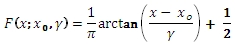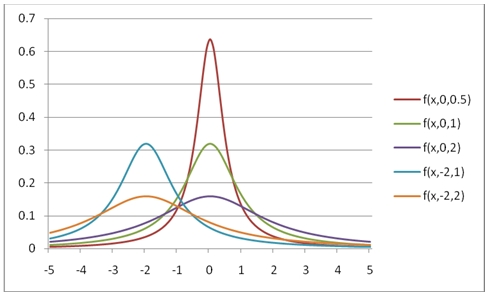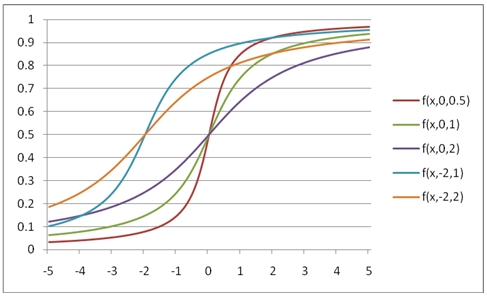# SQL Server Cauchy distribution function

CAUCHY

Updated: 31 July 2010

Use CAUCHY to calculate the probability density function or the lower cumulative distribution function of the CAUCHY distribution.

The formula for the probability density function is:The lower cumulative distribution function is:Syntax
SELECT [wctStatistics].[wct].[CAUCHY] (
<@X, float,>
,<@A, float,>
,<@B, float,>
,<@Cumulative, bit,>)
Arguments
@X
is the value to be evaluated. @X is an expression of type float or of a type that implicitly converts to float
@A
is the location parameter. @A is an expression of type float or of a type that implicitly converts to float
@B
is the shape parameter. @B is an expression of type float or of a type that implicitly converts to float
@Cumulative
is a logical value that determines if the probability density function ('False', 0) or the cumulative distribution function ('True', 1) is being calculated. @Cumulative is an expression of type bit or of a type that implicitly converts to bit

Return Types
float
Remarks
·         @B must be greater than zero (B > 0).
·         The upper cumulative distribution function is equal to 1 –CAUCHY(@X,@A,@B,'True').
Examples
Calculate the probability density function:
SELECT wct.CAUCHY(1,0,1,'False')
This produces the following result
----------------------
0.159154943091895

(1 row(s) affected)
You can use the SeriesFloat function from the XLeratorDB/math library to generate a dataset which can be pasted into EXCEL to generate a graph of the probability density function.
SELECT SeriesValue
,wct.CAUCHY(SeriesValue, 0, 0.5, 'False') as [f(x,0,0.5)]
,wct.CAUCHY(SeriesValue, 0, 1, 'False') as [f(x,0,1)]
,wct.CAUCHY(SeriesValue, 0, 2, 'False') as [f(x,0,2)]
,wct.CAUCHY(SeriesValue, -2, 1, 'False') as [f(x,-2,1)]
,wct.CAUCHY(SeriesValue, -2, 2, 'False') as [f(x,-2,2)]
FROM wct.SeriesFloat(-5, 5, .1,NULL,NULL)
This is an EXCEL-generated graph of the resultsCalculate the lower cumulative distribution function:
SELECT wct.CAUCHY(1,0,1,'True')
This produces the following result
----------------------
.75

(1 row(s) affected)
You can use the SeriesFloat function from the XLeratorDB/math library to generate a dataset which can be pasted into EXCEL to generate a graph of the cumulative distribution function.
SELECT SeriesValue
,wct.CAUCHY(SeriesValue, 0, 0.5, 'True') as [f(x,0,0.5)]
,wct.CAUCHY(SeriesValue, 0, 1, 'True') as [f(x,0,1)]
,wct.CAUCHY(SeriesValue, 0, 2, 'True') as [f(x,0,2)]
,wct.CAUCHY(SeriesValue, -2, 1, 'True') as [f(x,-2,1)]
,wct.CAUCHY(SeriesValue, -2, 2, 'True') as [f(x,-2,2)]
FROM wct.SeriesFloat(-5, 5, .1,NULL,NULL)
This is an EXCEL-generated graph of the resultsCalculate the upper cumulative distribution function:
SELECT 1 - wct.CAUCHY(1,0,1,'True')
This produces the following result
----------------------
.25

(1 row(s) affected)

### SupportCopyright 2008-2023 Westclintech LLC         Privacy Policy        Terms of Service